﻿ Conjugate Gradient Algorithm in the Four-Dimensional Variational Data Assimilation System in GRAPES
 J. Meteor. Res.2018, Vol. 32Issue (6): 974-984PDF
http://dx.doi.org/10.1007/s13351-018-8053-2
The Chinese Meteorological Society
0

#### Article Information

LIU, Yongzhu, Lin ZHANG, and Zhihua LIAN, 2018.
Conjugate Gradient Algorithm in the Four-Dimensional Variational Data Assimilation System in GRAPES. 2018.
J. Meteor. Res., 32(6): 974-984
http://dx.doi.org/10.1007/s13351-018-8053-2

### Article History

Received April 8, 2018
in final form August 20, 2018
Conjugate Gradient Algorithm in the Four-Dimensional Variational Data Assimilation System in GRAPES
Yongzhu LIU, Lin ZHANG, Zhihua LIAN
National Meteorological Center, Beijing 100081
ABSTRACT: Minimization algorithms are singular components in four-dimensional variational data assimilation (4DVar). In this paper, the convergence and application of the conjugate gradient algorithm (CGA), which is based on the Lanczos iterative algorithm and the Hessian matrix derived from tangent linear and adjoint models using a non-hydrostatic framework, are investigated in the 4DVar minimization. First, the influence of the Gram-Schmidt orthogonalization of the Lanczos vector on the convergence of the Lanczos algorithm is studied. The results show that the Lanczos algorithm without orthogonalization fails to converge after the ninth iteration in the 4DVar minimization, while the orthogonalized Lanczos algorithm converges stably. Second, the convergence and computational efficiency of the CGA and quasi-Newton method in batch cycling assimilation experiments are compared on the 4DVar platform of the Global/Regional Assimilation and Prediction System (GRAPES). The CGA is 40% more computationally efficient than the quasi-Newton method, although the equivalent analysis results can be obtained by using either the CGA or the quasi-Newton method. Thus, the CGA based on Lanczos iterations is better for solving the optimization problems in the GRAPES 4DVar system.
Key words: numerical weather prediction     Global/Regional Assimilation and Prediction System     four-dimensional variation     conjugate gradient algorithm     Lanczos algorithm
1 Introduction

The application of high-resolution data assimilation constitutes a mainstream technology for improving numerical weather prediction (NWP) models. Variational data assimilation, which is used to solve analysis problems by minimizing a given cost function, is the best way to estimate model initial conditions by accurately combining observation and background fields (Rabier, 2005; Bannister, 2017). Three-dimensional variational data assimilation (3DVar) was widely used in NWP centers during the twentieth century (Courtier et al., 1998; Rabier et al., 1998; Lorenc et al., 2000). However, 3DVar erroneously assumes that observations acquired at different times are taken at the same time within the assimilation window. To overcome the shortcomings of 3DVar, four-dimensional variational data assimilation (4DVar) seeks an optimal balance between observations scattered through time and space over a finite 4D analysis volume with priori information; consequently, 4DVar is able to closely fit both observations and a priori initial estimates to generate the optimal initial conditions for NWP models (Thépaut et al, 1993; Courtier et al., 1994). For more than a decade, 4DVar has been the most successful data assimilation method for global NWP models; it has been used by many of the main global NWP centers, such as the ECMWF (Rabier et al., 2000), the French national meteorological service Météo-France (Janisková et al., 1999), the Met Office (Rawlins et al., 2007), and the meteorological service of Canada (Laroche et al., 2007). In recent years, some new 4DVar methods for global NWP models have emerged, including the ensemble-based 4DVar technique (Liu and Xiao, 2013) and hybrid 4DVar that adds flow-dependent ensemble covariance to traditional incremental 4DVar, for example, the ensemble data assimilations at ECMWF (Isaksen et al., 2010) and the hybrid-4DVar method employed at the Met Office (Clayton et al. 2013Lorenc et al., 2015).

Variational data assimilation is a solution to large-scale unconstrained optimization problems. The cost function measuring the misfit between the background and the observations is first defined, and the optimal values are then determined by using various large-scale unconstrained minimization algorithms. Variational data assimilation techniques, especially 4DVar approaches based on the tangent linear model and adjoint model, are computationally expensive; thus, the development of a robust and efficient minimization algorithm is crucial (Fisher, 1998; Gürol et al., 2014). Two common minimization algorithms used in 4DVar systems are the conjugate gradient algorithm (CGA; Fisher, 1998) and quasi-Newton methods, including the limited-memory quasi-Newton method (i.e., the limited-memory Broyden–Fletcher–Goldfarb–Shanno, L-BFGS; Liu and Nocedal, 1989) and the truncated Newton method (Nash, 1984). Just as the L-BFGS method attempts to combine the modest storage and computational requirements of CGA methods with the convergence properties of standard quasi-Newton methods, truncated Newton methods attempt to retain the rapid (quadratic) convergence rate of classic Newton methods while making the storage and computational requirements feasible for large sparse matrices (Zou et al., 1993). Zou et al. (1993) compared the L-BFGS method with two truncated Newton methods on several test problems, including problems in meteorology and oceanography; their results confirmed that the L-BFGS seems to be the most efficient approach and is a particularly robust and user-friendly technique. Navon and Legler (1987) compared a number of different CGA and L-BFGS approaches for problems in meteorology and concluded that the L-BFGS is the most adequate for large-scale unconstrained minimization algorithms in meteorology. Furthermore, Fisher (1998) compared different CGA and truncated Newton methods in the ECMWF, and they concluded that the CGA was the most adequate for their 4DVar system. Therefore, the preconditioned CGA is used in the operational 4DVar system of ECMWF (Trémolet, 2007).

The 3DVar operational assimilation system is employed in the Global/Regional Assimilation and Prediction System (GRAPES; Shen et al., 2017) with the L-BFGS minimization algorithm (Xue et al., 2008). The GRAPES dynamical core uses a non-hydrostatic framework with two-time-layer semi-implicit and semi-Lagrangian discretization and employs a latitude–longitude grid with the staggered Arakawa C grid for spatial discretization. A 4DVar system has been developed in the GRAPES to improve its operational prediction quality by using the non-hydrostatic tangent linear and adjoint models, which were developed for the GRAPES global data assimilation system (Liu et al., 2017). The L-BFGS method is currently applied to the GRAPES 4DVar system, but its low convergence rate leads to a low computational efficiency (Zhang and Liu, 2017). In this paper, to select a robust and efficient minimization algorithm for the GRAPES 4DVar system, the convergence of the CGA is thoroughly examined, and the CGA is compared with the L-BFGS method in the GRAPES 4DVar scheme.

This paper is organized as follows. The data and methods are described in Section 2. Section 3 investigates the convergence of the CGA, and some results of the CGA in the GRAPES 4DVar system based on the numerical experiments are presented in Section 4. The conclusions and outlook are presented in Section 5.

2 Data and methods 2.1 Incremental 4DVar

Incremental formulation is commonly used in variational data assimilation systems (Courtier et al., 1994; Trémolet, 2007). The incremental scheme offers two main advantages: 1) the tangent linear model and adjoint model can be used with a reduced resolution during minimization, largely reducing the computational cost of 4DVar; 2) the cost function becomes strictly quadratic, and thus, the convergence rate of the minimization can be greatly improved (Fisher, 1998). The incremental formulation scheme includes two components, namely, the inner loop and the outer loop. The outer loop utilizes the initial estimate of the atmospheric state as the initial condition of the forecast model and obtains the model trajectory within the assimilation time windows; this trajectory is then used to calculate the observational increments within the time windows. The purpose of the inner loop is to solve the minimization problem by an iterative algorithm for the variational data assimilation.

In the 4DVar incremental formulation, the first-order approximation of the cost function $J$ is written as the control variable $\delta {x}$ (Courtier et al., 1994; Fisher, 1998; Trémolet, 2007):

 $\begin{split} J \left( {\delta {x}} \right) & = \frac{1}{2}\delta {{x}^{\rm{T}}}{{{B}}^{{ - 1}}}\delta {x} + \frac{1}{2}{\sum\nolimits_{{i} = 0}^{n} {\left( {{{{H}}_{i} }{{{L}}_{0 \to {i}}}\delta {x} - {{d}_{i}}} \right)} ^{\rm{T}}}{{R}}_{i}^{{ - 1}}\left( {{{{H}}_{i} }{{{L}}_{0 \to {i}}}\delta {x} - {{d}_{i}}} \right) \\& =\frac{1}{2}\delta {{x}^{\rm{T}}}\left( {{{{B}}^{ - 1}} + \sum\nolimits_{{i} = 0}^{n} {{{L}}_{{i} \to 0}^{\rm{T}}{{H}}_{i}^{\rm{T}}{{R}}_{i}^{{ - 1}}{{{H}}_{i}}{{{L}}_{0 \to {i}}}} } \right)\delta {x} - \delta {{x}^{\rm{T}}}\sum\nolimits_{{i} = 0}^{n} {{{L}}_{{i} \to 0}^{\rm{T}}{{H}}_{i}^{\rm{T}}{{R}}_{i}^{{ - 1}}{{d}_{i}}} + \frac{1}{2}{d}_{i}^{\rm{T}}{{R}}_{i}^{{ - 1}}{{d}_{i}}\end{split},$ (1)

where $\delta {x}$ is the departure from the background $\left({\delta {x} = {x} - {{x}_{\rm b}}} \right)$ , which will be the analysis increment; ${x}$ is the model state at time t0; ${{x}_{\rm b}}$ is the background state at time t0; B is the background error covariance matrix; ${{{R}}_{i}}$ is the observational error covariance matrix at time ti; ${{{H}}_i} = \partial {\mathcal{H}_i}/\partial {x}$ is the linearized observation operator of the nonlinear observation operator ${\mathcal{H}_i}$ at time ti; ${{{L}}_{0 \to {i}}} =$ ${{\partial {\mathcal{M}_{0 \to {i}}}}/{\partial {x}}}$ is the tangent linear model of the nonlinear model ${\mathcal{M}_{0 \to {i}}}$ integrated from time t0 to time ti; and ${{L}}_{{i} \to 0}^{\rm{T}}$ is the corresponding adjoint operator of ${{{L}}_{0 \to {i}}}$ that constitutes backward integration from time ti to time t0; ${{d}_{i}} = {{o}_{i}} - {{{H}}_i}\partial {\mathcal{M}_{0 \to {i}}}({{x}_{\rm b}})$ represents the observational increment at time ti; ${{o}_{i}}$ is the observation at time ti. The solution of the adjoint operator can be coded from the corresponding tangent linear model code, and it does not require deriving the adjoint equations analytically (Talagrand and Courtier, 1987).

To solve the minimization problem of Eq. (1), the gradient of the control variable δx is calculated with the following equation (Courtier et al., 1994; Fisher, 1998):

 $\begin{split}\nabla {J}\left({\delta {x}} \right) = & \left({{{{B}}^{ - 1}} + \sum\nolimits_{{i} = 0}^{n} {{{L}}_{{i} \to 0}^{\rm{T}}{{H}}_{i}^{\rm{T}}{{R}}_{i}^{ - 1}{{{H}}_{i}}{{{L}}_{0 \to {i}}}} } \right)\delta {x} \\ - & \sum\nolimits_{{i} = 0}^{n} {{{L}}_{{i} \to 0}^{\rm{T}}{{H}}_{i}^{\rm{T}}{{R}}_{i}^{ - 1}{{d}_{i}}}, \end{split}$ (2)

where the minimization of the cost function can be obtained by minimization algorithms such as the Newton method or CGA. The second partial derivative of ${J}\left({\delta {x}} \right)$ , the Hessian matrix, is denoted ${J''}$ and is calculated as follows (Courtier et al., 1994; Fisher, 1998):

 ${J''} = {{{B}}^{ - 1}} + \sum\nolimits_{{i} = 0}^{n} {{{L}}_{{i} \to 0}^{\rm{T}}{{H}}_{i}^{\rm{T}}{{R}}_{i}^{ - 1}{{{H}}_{i}}{{{L}}_{0 \to {i}}}} .$ (3)

Thus, the solution of Eq. (2) is equal to the solution of the system of linear equations ${J''}\delta {x} = {b}$ , where ${b} = \displaystyle\sum\nolimits_{{i} = 0}^{n}$ ${{{L}}_{{i} \to 0}^{\rm{T}}{{H}}_{i}^{\rm{T}}{{R}}_{i}^{ - 1}{{{H}}_{i}}{{{L}}_{0 \to {i}}}}$ .

Because B is usually a large sparse matrix and is nearly ill conditioned, it is difficult to solve the minimization problem in Eq. (1). To achieve acceptable convergence rates, it is necessary to perform some transforms and preconditioning for B.

In the GRAPES 4DVar system, the basic atmospheric state variables x are the two wind vectors (denoted by u and v), the relative humidity q, and the non-dimensional pressure as an independent variable (denoted by $\Pi$ ), which is the analysis variable that represents the quality field, instead of potential temperature (denoted by θ). Thus, the analysis increment is $\delta {x} = {\left({\delta {u}, \delta {v}, \delta \Pi } \right)^{\rm{T}}}$ , which can be transformed into a new vector $\delta {{x}_{u}} = {\left({\delta \psi, \delta {\chi _{u}}, \delta {\Pi _{u}}} \right)^{\rm{T}}}$ , $\delta {x} = \mathcal{P}\delta {{x}_{u}}$ , where $\mathcal{P}$ is a physical balance transformation operator (Xue et al., 2008).

Therefore, the background error covariance matrix B can be split into three independent blocked matrices ${{B}} = \mathcal{P}{{{B}}_{u}}{\mathcal{P}^{ - 1}}$ , thereby reducing the scale of the matrix computation. This method of preconditioning through a change in the variable ${\left({{{{B}}_{u}}} \right)^{{1/2}}}$ is currently used in the GRAPES 4DVar system. Introducing a new control variable w in the cost function, the preconditioning transform of the variable $\delta {x}$ is expressed as $\delta {x} = \mathcal{P}\delta {{x}_{u}} =$ $\mathcal{P}{\Sigma _{u}}\mathbb{U}{w}$ , where ${{{B}}_{u}} = {\Sigma _{u}}\mathbb{U}\mathbb{U}{\Sigma _{u}}$ . Therefore, Eq. (1) can be expressed by using the control variable w:

 $\begin{split}J\left({w} \right) = & \frac{1}{2}{{w}^{\rm{T}}}{w} + \frac{1}{2}{\sum\nolimits_{{i} = 0}^{n} {\left({{{{H}}_{i} }{{{L}}_{0 \to {i}}}\mathcal{P}{\Sigma _{u}}\mathbb{U}{w} - {{d}_{i}}} \right)} ^{\rm{T}}}\\ & \cdot {{R}}_{i}^{ - 1}\left({{{{H}}_{i} }{{{L}}_{0 \to {i}}}\mathcal{P}{\Sigma _{u}}\mathbb{U}{w} - {{d}_{i}}} \right).\end{split}$ (4)
2.2 The L-BFGS and CGA in GRAPES 4DVar

The L-BFGS algorithm (Appendix A) in the GRAPES 4DVar system uses the estimation to the inverse Hessian matrix to guide its search through the variable space. For the L-BFGS in the GRAPES 4DVar scheme, the initial Hessian matrix is the identity matrix, and the number of iterations m insomuch that the m previous values sk and zk are stored to compute the approximation of the inverse Hessian matrix is 12.

The CGA based on the Lanczos iteration (Appendix B) in GRAPES 4DVar is mainly applied to solve large sparse, symmetric, positive definite linear equations (Paige and Saunders, 1982). With this combination, the orthogonalization of the Lanczos algorithm can sufficiently overcome the instability of the CGA in providing practical solutions to the above equations.

The Hessian matrix ${J''}$ in Eq. (3) is a sparse, real, symmetric, positive definite matrix that can be computed by using B, R, H, L, and LT. The convergence efficiency of the inner loop minimization of 4DVar is largely determined by the shape of the Hessian matrix, and the computational efficiency largely depends on that of the tangent linear model L and the adjoint model LT as well as the number of iterations in the minimization. Therefore, this approach effectively improves the computational efficiency of the 4DVar minimization by choosing an efficient iterative minimization algorithm.

2.3 Orthogonalization of the CGA

Rounding errors greatly affect the behavior of the Lanczos iteration for a practical minimization problem (Paige, 1970). For a 4DVar system in particular, there are often some computational errors from the tangent model and adjoint model of the Hessian matrix ${J''}$ as well as rounding errors from the iterations. These errors lead to a quick loss of orthogonality in the Lanczos vectors ${{q}_{k}}$ in addition to the problem of “ghost” eigenvalues during the Lanczos iterations. Moreover, there are multiple eigenvalues of ${{{T}}_{k}}$ that correspond to simple eigenvalues of the Hessian matrix ${J''}$ ; this results in additional iterations and convergence failure. Thus, the application of the Lanczos algorithm can easily cause numerical instabilities in the solutions of large symmetric matrices. However, this issue can be overcome by conducting Gram-Schmidt orthogonalization on the Lanczos vectors (Paige, 1970), which is conducted primarily by three methods as follows:

(1) Full orthogonalization (Paige, 1970). This process conducts Gram-Schmidt orthogonalization to make the Lanczos vector ${{q}_{{k} + 1}}$ orthogonal to all of the previously computed Lanczos vectors. In detail, the Gram-Schmidt orthogonalization is applied to the residual vector ${{r}_{{k + }{1}}}$ derived from the third step of the Lanczos algorithm [Eq. (B4)] and the Lanczos vector groups $\left({{{q}_{1,\cdots,}}{{q}_{k}}} \right)$ , i.e., ${{r}_{k + 1}} = {{r}_{k + 1}} - \displaystyle\sum\nolimits_{{i} = 1}^{k} {\left\langle {{{r}_{k + 1}}, {{q}_{i}}} \right\rangle } {{q}_{i}}$ . Thus, the Lanczos vector ${{q}_{{k} + 1}}$ will be orthogonal to the previously computed Lanczos vectors $\left({{{q}_{1,\cdots,}}{{q}_{k}}} \right)$ .

(2) Partial orthogonalization (Simon, 1984). Consequently, instead of orthogonalizing ${{q}_{{k} + 1}}$ against all the previously computed Lanczos vectors, the same effect can be achieved by orthogonalizing ${{q}_{{k} + 1}}$ against the previously computed Lanczos vectors that are not orthogonal to ${{q}_{{k} + 1}}$ . The detailed steps are similar to those in the full orthogonalization method. However, this method reduces the number of orthogonalized inner products and therefore improves the computational efficiency.

(3) Selective orthogonalization (Parlett and Scott, 1979). The method is similar to partial orthogonalization but orthogonalizing ${{q}_{{k} + 1}}$ against the much smaller set of converged eigenvectors of the Hessian matrix ${J''}$ . This method can avoid some calculations of repeated eigenvalues, reduce the additional Lanczos iterations, and improve the computational efficiency. However, extra space is needed to store the eigenvectors.

The CGA has been successfully applied in the 4DVar system of ECMWF (Fisher, 1998; Trémolet, 2007). However, there are many differences between the GRAPES and ECMWF 4DVar systems. First, the ECMWF tangent linear model and adjoint model use a hydrostatic framework with spectral and reduced grids, while those in GRAPES employ a non-hydrostatic framework with a latitude–longitude grid. Especially in polar regions, the denser grid distribution of GRAPES adds a gradient sensitivity computed by the adjoint model, leading to an increase in the condition number of the Hessian matrix ${J''}$ , thereby affecting the convergence rate. Second, the state variables of assimilation and the tangent linear model variables are the same as those in the ECMWF 4DVar system. However, there is a variable physical transform between the tangent linear model and the assimilation system (see Section 2.1).

3 Data and experiment

To further analyze the effectiveness of the CGA in a practical 4DVar system, we conduct one cycling assimilation experiment for a month. The time ranges from 0900 UTC 1 June to 0900 UTC 1 July 2016. The data used for the assimilation include conventional Global Telecommunication System (GTS) observations, including temperature, wind and relative humidity data derived from sounding, pressure data from ships and the Synoptic Ocean Prediction (SYNOP) experiment, and wind data from pilot readings, in addition to data from satellite-based platforms, such as the NOAA-15 Advanced Microwave Sounding Unit-A (AMSUA), NOAA-18 AMSUA, NOAA-19 AMSUA, MetOp-A AMSUA, MetOp-B AMSUA, National Polar-orbiting Partnership (NPP) Advanced Technology Microwave Sounder (ATMS) AMSUA, SeaWinds scatterometer, and Global Navigation Satellite System (GNSS) radio occultations. Satellite observations compose approximately 70% of the total observations. The assimilation window is 6 h, and the observational interval is 30 min. The horizontal resolution of the outer loop is 0.5°, and the model time step is 450 s. The horizontal resolution of the inner loop is 1.5°, and the model time step is 900 s. The number of vertical levels is 60, and the maximum number of iterations is 70 in the 4DVar minimization.

The following linearized physical processes are used in this 4DVar experiment: two dry linearized physical processes (vertical diffusion and subgrid-scale orographic effects) to improve the representation of perturbed fields in the tangent linear model (Liu et al., 2017), and two newly developed moist linearized physical parameterizations consisting of deep cumulus convection based on a new simplified Arakawa-Shubert scheme (Han and Pan, 2006) and the large-scale cloud and precipitation scheme described in Tompkins and Janisková (2004). The experimental environment is based on the high-performance computer (Sugon PI) at the China Meteorological Administration. In total, 256 CPU cores are used in these experiments. Two configurations of 4DVar experiments are tested:

(1) CGA experiments, in which the CGA is used for minimization in the 4DVar system; and

(2) L-BFGS experiments, in which the L-BFGS is used for minimization in the 4DVar.

4 Results of the CGA in 4DVar

We perform numerical experiments on the GRAPES 4DVar system to investigate the convergence of the CGA therein. The experimental configuration is the same as that in the batch experiments in Section 3, which begin at 0900 UTC 1 June 2016, and the number of iterations is 120 in the minimization. Then, the numerical stability of the Lanczos algorithm in the 4DVar is tested against the four orthogonalization schemes described in Section 2.3: full orthogonalization, partial orthogonalization, selective orthogonalization, and without orthogonalization.

4.1 Orthogonalization analysis of the CGA

The convergence of the gradient norm $\left\| {\nabla {J}\left({\delta {x}} \right)} \right\|$ [Eq. (2)] with the non-orthogonalized Lanczos vector ${{q}_{k}}$ is shown in Fig. 1. The gradient norm fails to converge starting at the 9th iteration, which is partly the result of computational errors. As the iteration continues, the reduced orthogonality of the Lanczos vector ${{q}_{k}}$ gives rise to a higher gradient norm. However, the convergence of the gradient norm is much better after performing full orthogonalization on the Lanczos vector ${{q}_{k}}$ (blue dashed line in Fig. 1). In addition, the results of the first nine iterations are the same as those without orthogonalization. This outcome indicates that the orthogonalization on Lanczos vector ${{q}_{k}}$ does not change the iteration results of the Lanczos algorithm when the effect of the computational errors is small, while the orthogonalization on the Lanczos vector ${{q}_{k}}$ can effectively eliminate the effects of computational errors, leading to the stable convergence of the Lanczos algorithm when the computational errors become larger. Further, the results of partial orthogonalization (red dotted line in Fig. 1) on the Lanczos vector ${{q}_{k}}$ are the same as those of full orthogonalization, and selective orthogonalization also produces the same results as full orthogonalization.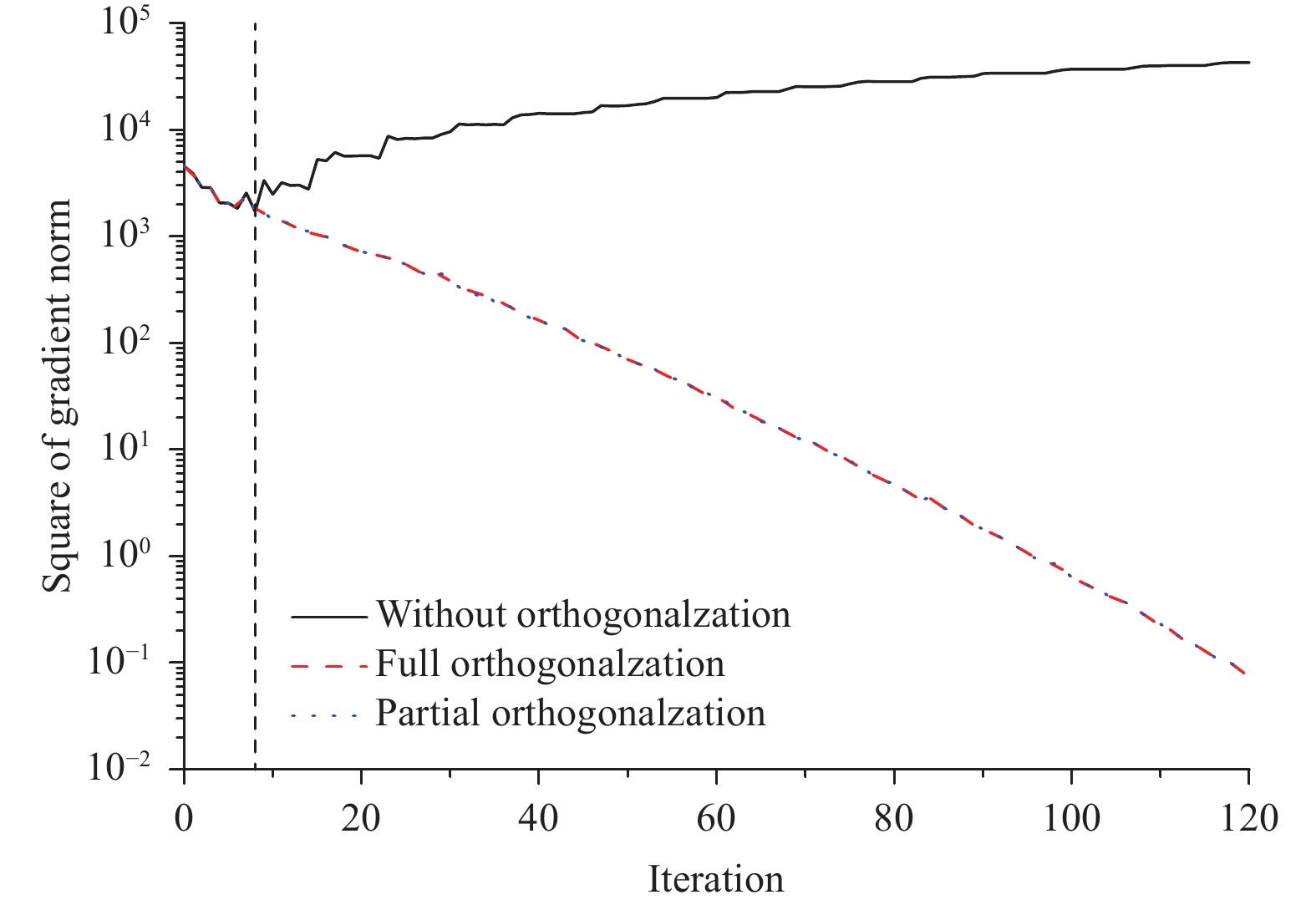Figure 1 Convergence of the conjugate gradient norm as a function of the number of iterations for a 4DVar cost function. The vertical axis is the square of the gradient norm, and it denotes the difference between the control vector at a given iteration and its 120th iteration (black solid line: without orthogonalization; red dashed line: full orthogonalization; blue dotted line: partial orthogonalization; vertical dotted line shows that the gradient norm fails to converge starting at the 9th iteration without orthogonalization).

The eigenvalue distribution of the Hessian matrix ${J''}$ under different orthogonalization methods is illustrated in Fig. 2. The eigenvalue distribution without orthogonalization on the Lanczos vector ${{q}_{k}}$ is indicated by the solid line (Fig. 2). There are 53 convergent eigenvalues in total (circles on the solid line in Fig. 2); many repeated eigenvalues are associated with redundant iterations due to the loss of orthogonality of the Lanczos vector ${{q}_{k}}$ during the iterations. This Lanczos algorithm is numerically unstable in the 4DVar minimization. The red dashed line in Fig. 2 shows the eigenvalue distribution with full orthogonalization performed on the Lanczos vector ${{q}_{k}}$ . Moreover, the number of convergent eigenvalues (triangles on the dashed line in Fig. 2) is 53, but these eigenvalues are no longer repeated. This result implies that the Lanczos algorithm is stable in the 4DVar minimization after conducting full orthogonalization on the Lanczos vector ${{q}_{k}}$ . The number of convergent eigenvalues (blue dotted line in Fig. 2) with partial orthogonalization applied to the Lanczos vector ${{q}_{k}}$ is 49, which is 4 fewer than that with full orthogonalization. However, the eigenvalue distribution with partial orthogonalization is generally similar to that with full orthogonalization. Similarly, the eigenvalue distribution with selective orthogonalization is also generally similar to that with full orthogonalization. Therefore, the Lanczos algorithm is stable in the 4DVar minimization using full orthogonalization, partial orthogonalization, or selective orthogonalization.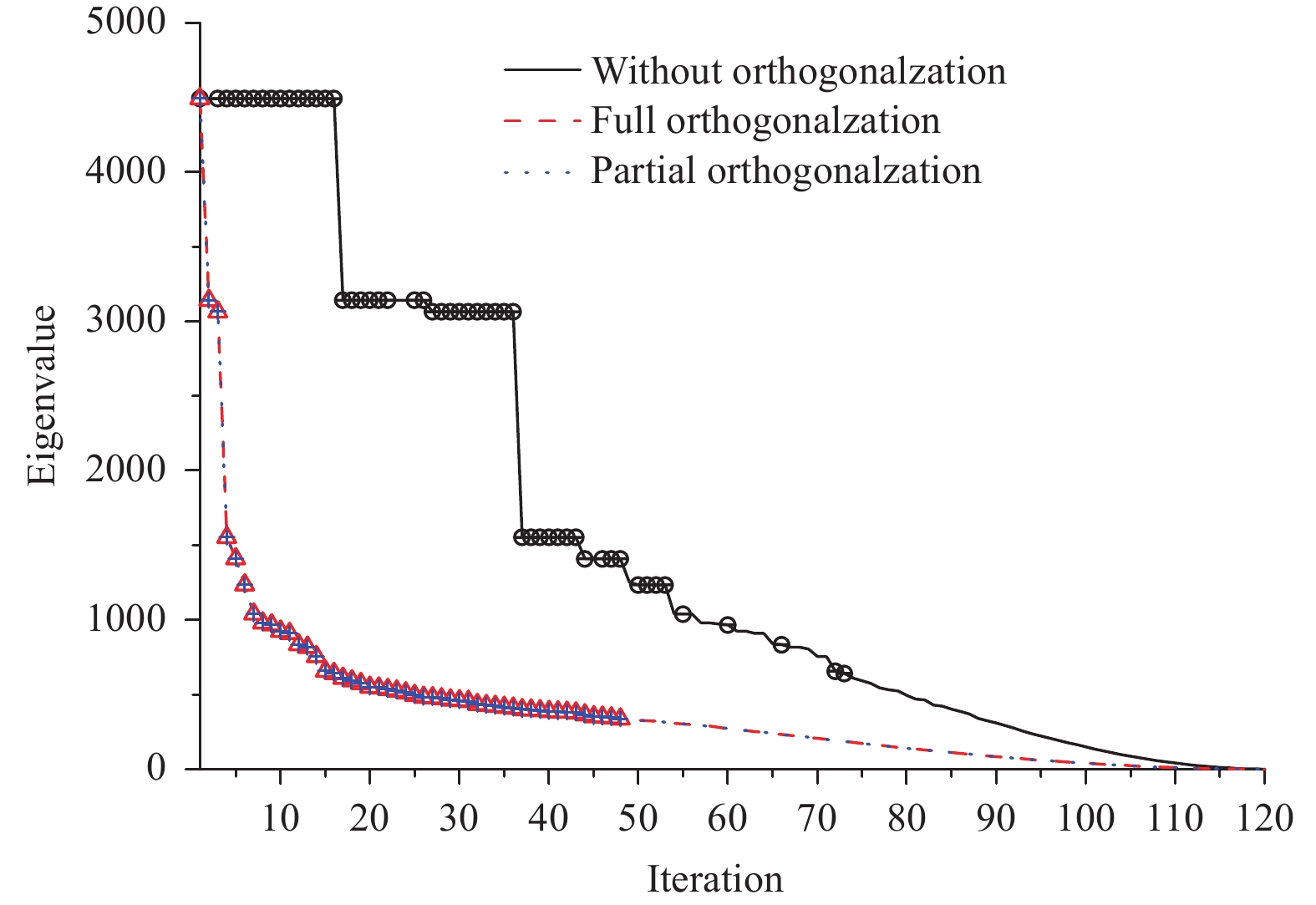Figure 2 Eigenvalue distribution of the Hessian matrix of a 4DVar minimization with different schemes of the orthogonalization of Lanczos vectors against the number of iterations (black solid line: without orthogonalization; red dashed line: full orthogonalization; blue dotted line: partial orthogonalization).
4.2 Convergence analysis of the CGA

In the 4DVar minimization, the convergence rate of the CGA depends on the eigenvalue distribution of the Hessian matrix ${J''}$ and the condition number κ (the ratio of the maximum eigenvalue to the minimum eigenvalue). The convergence estimation of the CGA, namely, the conjugated error $\left({{{e}_{j}} = \delta {x} - \delta {{x}_{j}}} \right)$ , is based on the norm of the Hessian matrix, and it satisfies the following (Paige, 1970; Fisher, 1998):

 $\left\| {{{e}_{j}}} \right\|_{{J''}}^2 \!=\! {\left({\delta {x} \!-\! \delta {{x}_{j}}} \right)^{\rm{T}}}{J''}\left({\delta {x} \!-\! \delta {{x}_{j}}} \right) \leqslant 2{\left({\frac{{\sqrt {\kappa } \!-\! 1}}{{\sqrt {\kappa } \!+\! 1}}} \right)^{j}}\left\| {{{e}_{0}}} \right\|_{{J''}}^2.$ (5)

Here, δx is the solution of the 4DVar minimization in Eq. (1) (the value of which is the estimated solution of the last iteration of the CGA), while $\delta {{x}_{j}}$ is the estimated solution at the jth iteration of CGA. According to Eq. (B8), the CGA should converge better than the linear algorithm. Moreover, the convergence can be improved by the pre-optimization step of reducing the condition number.

To explore the convergence of the CGA in the GRAPES 4DVar system, we conduct an assimilation experiment (beginning at 0900 UTC 1 June 2016) with 120 iterations of 4DVar minimization. The maximum (minimum) eigenvalue of the Hessian matrix ${J''}$ estimated by the CGA in the 4DVar minimization is 4492.1 (1.03). Per Eq. (5), the convergence rate ${{\left({\sqrt {\kappa } - 1} \right)}/{\left({\sqrt {\kappa } + 1} \right)}}$ estimated by the condition number is 0.970, and the upper bound on the convergence rate is expressed by the solid line in Fig. 3. This result implies that the convergence of the Hessian matrix is unsatisfactory. However, in a practical calculation of the 4DVar minimization based on the CGA, the Hessian norm of the true iteration error $\left\| {{{e}_{j}}} \right\|_{{J''}}^2$ decreases in magnitude from 103 to 10–2 after 120 iterations. The descent rate is clearly quicker than the convergence rate estimated by the condition number, which constitutes superlinear convergence. The above results are consistent with those based on the Integrated Forecasting System of ECMWF (Fisher, 1998).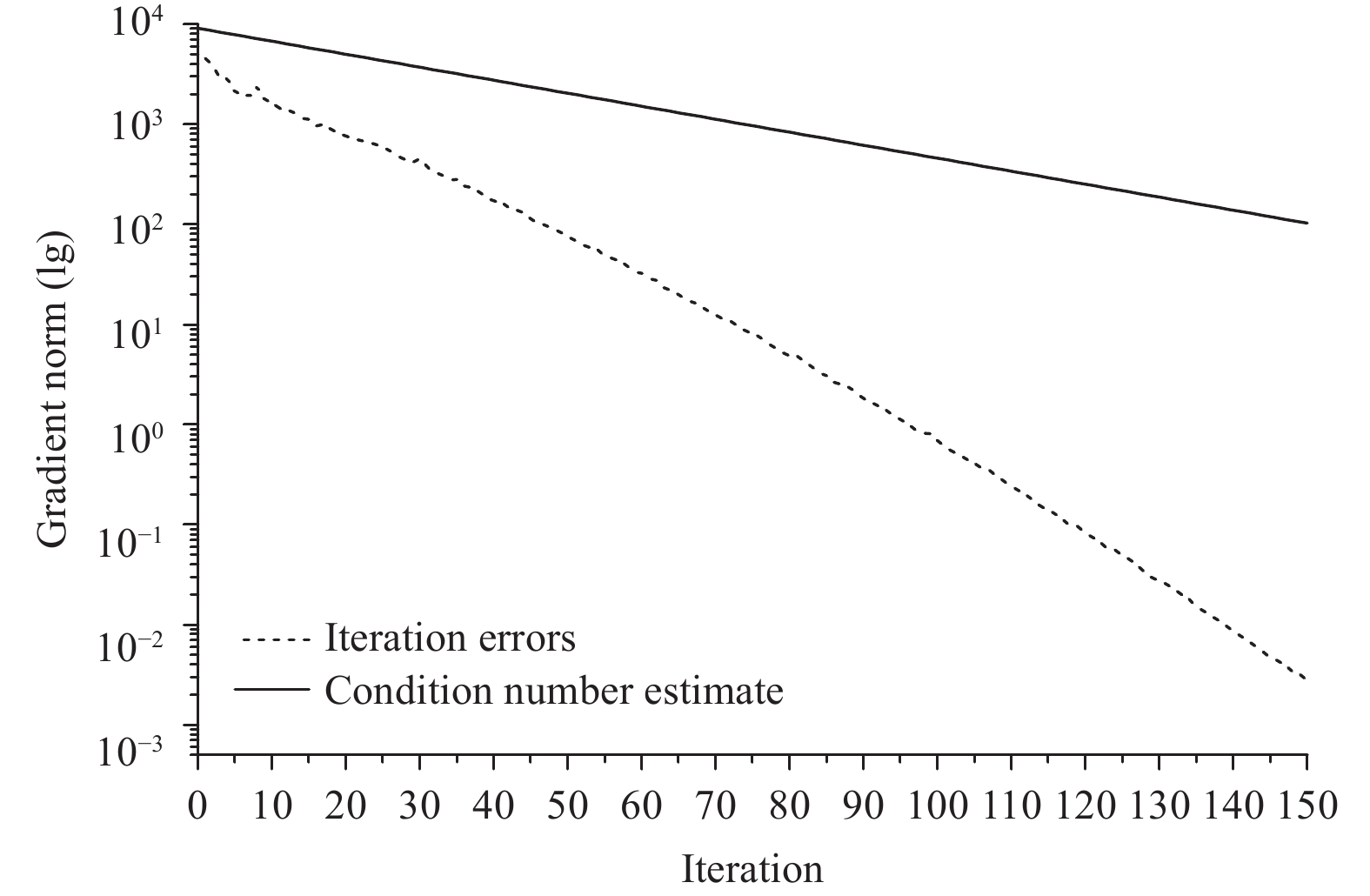Figure 3 Convergence of the CGA as a function of the number iterations for a 4DVar cost function. The dashed line is the square of the Hessian norm of the difference between the control vector and the value of the last iteration. The solid line is the upper bound of the convergence rate defined by Eq. (5).

In short, the Lanczos algorithm is numerically more stable in the 4DVar minimization if the Lanczos vector is orthogonal during the Lanczos iterations. Thus, performing Gram-Schmidt orthogonalization on the Lanczos vector is an effective way to ensure the numerical stability in the Lanczos algorithm. In this way, the convergence rate of 4DVar minimization is also improved. Considering the short computational time for orthogonalization in the whole 4DVar system, we exploit the Lanczos algorithm with full orthogonalization in the GRAPES 4DVar system to guarantee the orthogonality of the Lanczos vector.

5 Results of numerical experiments in 4DVar 5.1 Comparison of convergence with the L-BFGS

The CGA and quasi-Newton method are both quadratically convergent in theory and have the same quadratic termination property. However, these methods behave quite differently in practical applications, especially when applied to certain problems such as solving the minimization of 4DVar. To better compare the convergences of these two methods in 4DVar minimization problems, both cycling assimilation experiments begin on 10 June 2016. Recalculations are performed in the 4DVar experiments with the CGA using the background from the L-BFGS tests. The ratio of the root mean square of the gradient norm to its initial value is regarded as the convergence criterion (set as 0.03) during the minimization iterations. The maximum number of iterations is 70.

In the first dozen 4DVar minimization iterations in the four assimilation experiments, the gradient norms of the CGA and L-BFGS experiments both decrease with some oscillations, and a smaller amplitude is observed for the CGA experiments (Fig. 4). Then, the oscillation of the gradient norm for the CGA experiments becomes small, and these experiments satisfy the convergence criterion after approximately 40 iterations. However, the oscillation of the gradient norm for the L-BFGS experiments is still large, and the experiments do not converge after 70 iterations. In addition, the gradient norm in the L-BFGS experiments descends very slowly in the later stages, and the minimum is similar to that after approximately 40 iterations in the CGA experiments (dotted line in Fig. 4). The above results indicate that the convergence of the CGA is better than that of the L-BFGS in the 4DVar minimization. Moreover, the computational cost of the CGA is lower. Therefore, the CGA is preferable.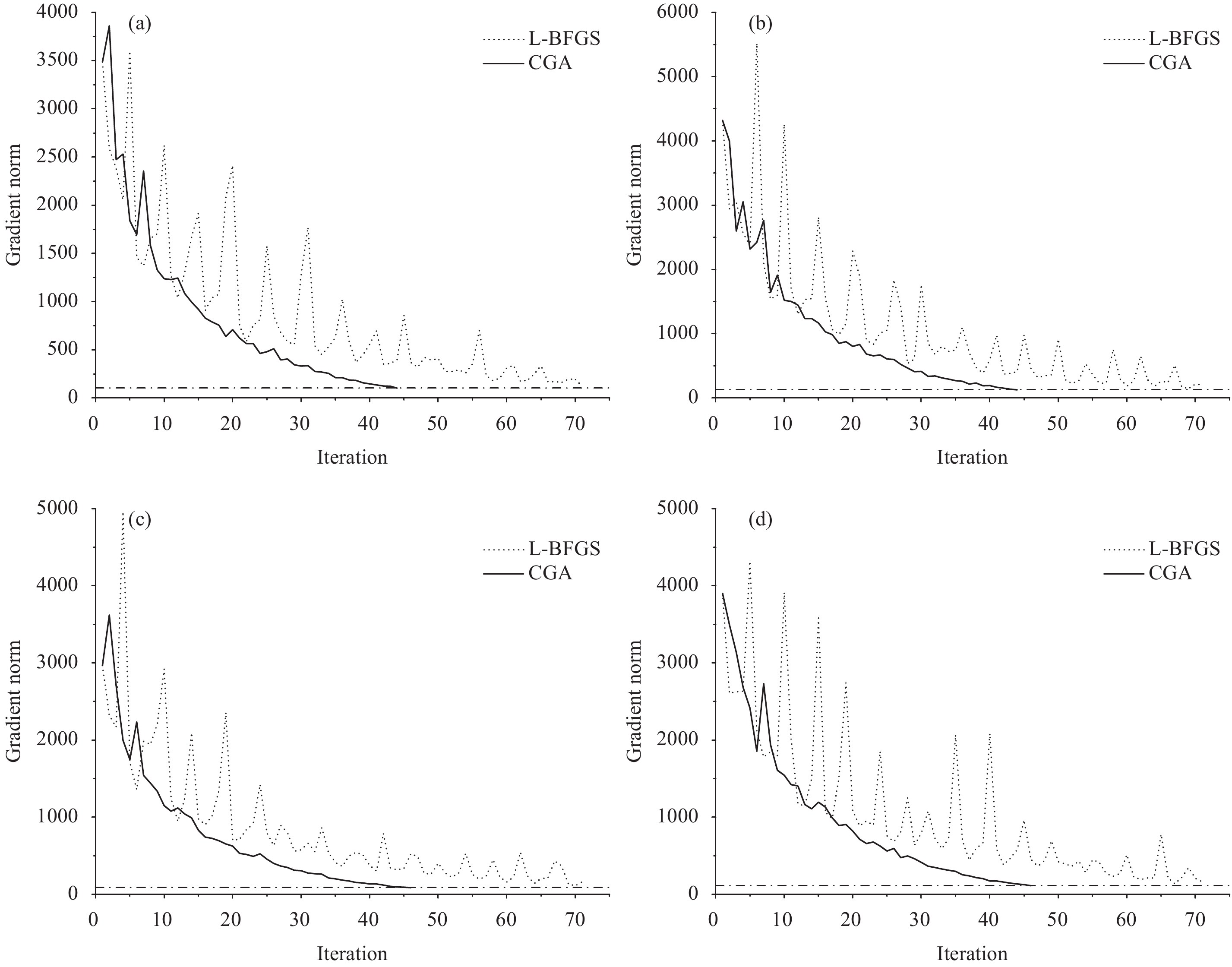Figure 4 Convergence of the Hessian norm for a 4DVar cost function under the GRAPES global 4DVar assimilation system starting at (a) 0300 UTC 10 June 2016, (b) 0900 UTC 10 June 2016, (c)1500 UTC 10 June 2016, and (d) 2100 UTC 10 June 2016 (solid line: CGA experiment; dotted line: L-BFGS experiment).

To better compare the convergences of the cost functions between the two sets of experiments, normalization is applied to the cost function to calculate the ratio of all the cost functions to the initial cost function within the iterations. The convergences of the cost functions for both the CGA experiments and the L-BFGS experiments on 20 June 2016 in the 4DVar cyclical assimilations are illustrated in Fig. 5. In the first twenty iterations, the descent rate of the cost functions of the CGA experiments are faster than those of the L-BFGS experiments. The convergence in the CGA experiments after 40 iterations is similar to that in the L-BFGS experiments after 70 iterations. Thus, the convergence rate of the CGA is much faster than that of the L-BFGS in the 4DVar minimization.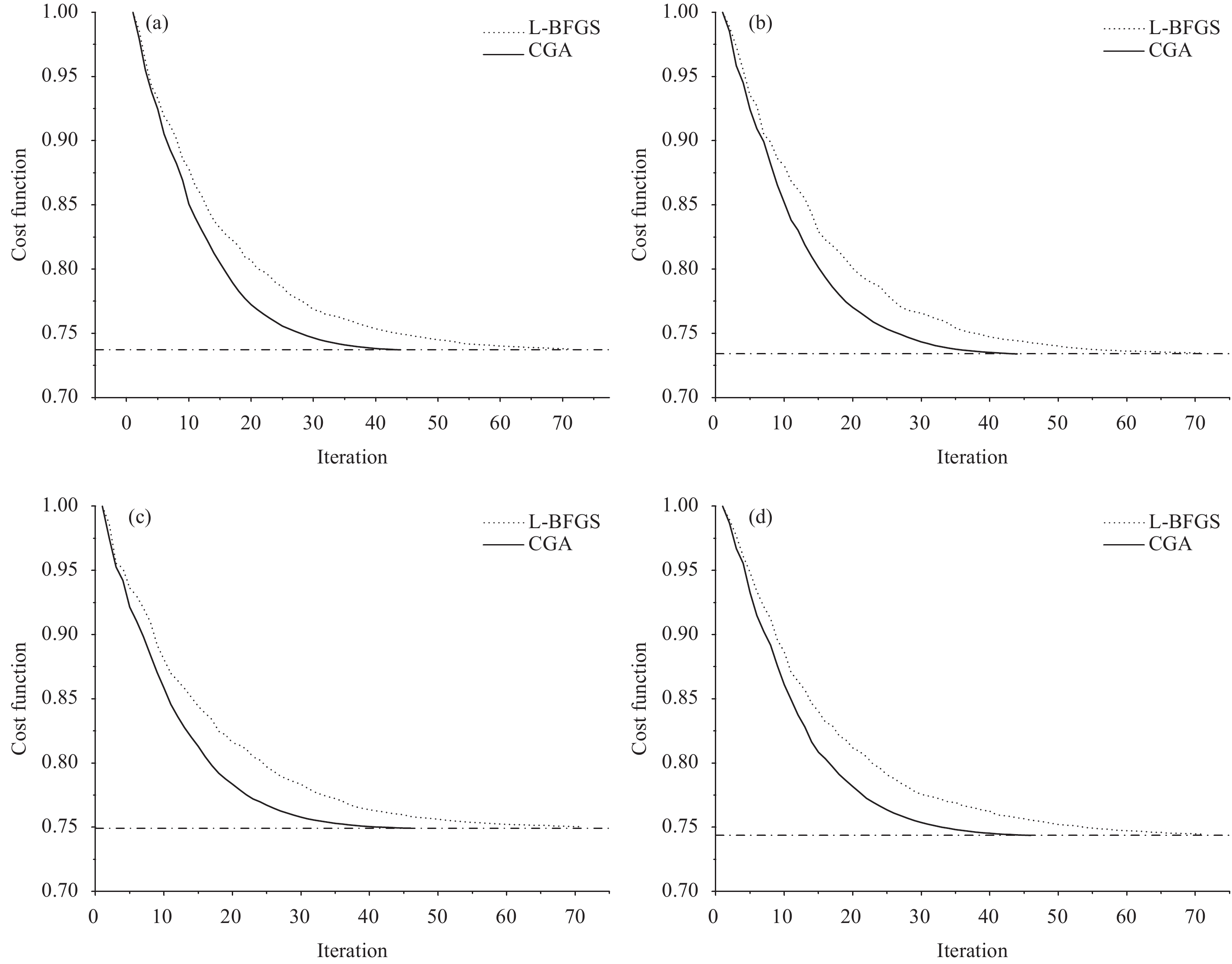Figure 5 Convergence of the 4DVar cost function under the GRAPES global 4DVar assimilation system starting at (a) 0300 UTC 20 June 2016, (b) 0900 UTC 20 June 2016, (c) 1500 UTC 20 June 2016, and (d) 2100 UTC 20 June 2016 (solid line: CGA experiment; dotted line: L-BFGS experiment; horizontal dashed line: final convergence rate of the cost function in the CGA experiment).
5.2 Computational efficiency

In the 4DVar minimization, the term ${J''}{{q}_{k}}$ should be calculated with the iteration of the tangent and adjoint models. Therefore, the computational cost, which is determined by the number of iterations, is very high. Hence, improving the convergence rate and reducing the number of iterations is an effective way to improve the computational efficiency of the 4DVar minimization.

The numbers of iterations and the calculation times for the 4DVar minimization in the 121 cyclical assimilation tests for both experimental sets are plotted in Fig. 6. The CGA experiments satisfy the requirement for convergence within a maximum of 70 iterations in the minimization. The average number of iterations to reach convergence is 37, and the average minimization time in these CGA experiments is 861 s. However, most of the L-BFGS experiments do not meet this convergence requirement within the maximum number of iterations because some of the cost functions in the iterations do not decrease when using the L-BFGS minimization, and thus, it is necessary to choose another descent direction for the L-BFGS experiments, which requires additional calculations. Thus, the number of iterations for some L-BFGS experiments is greater than the maximum number of iterations (70). Furthermore, the average number of iterations in these L-BFGS experiments is 68, and the average minimization time in these L-BFGS experiments is 1443 s. The average number of iterations in the CGA experiments is 32 less than that in the L-BFGS experiments, representing a 45% improvement in the computational efficiency. Hence, the CGA can largely improve the computational efficiency without affecting the convergence in the GRAPES 4DVar system.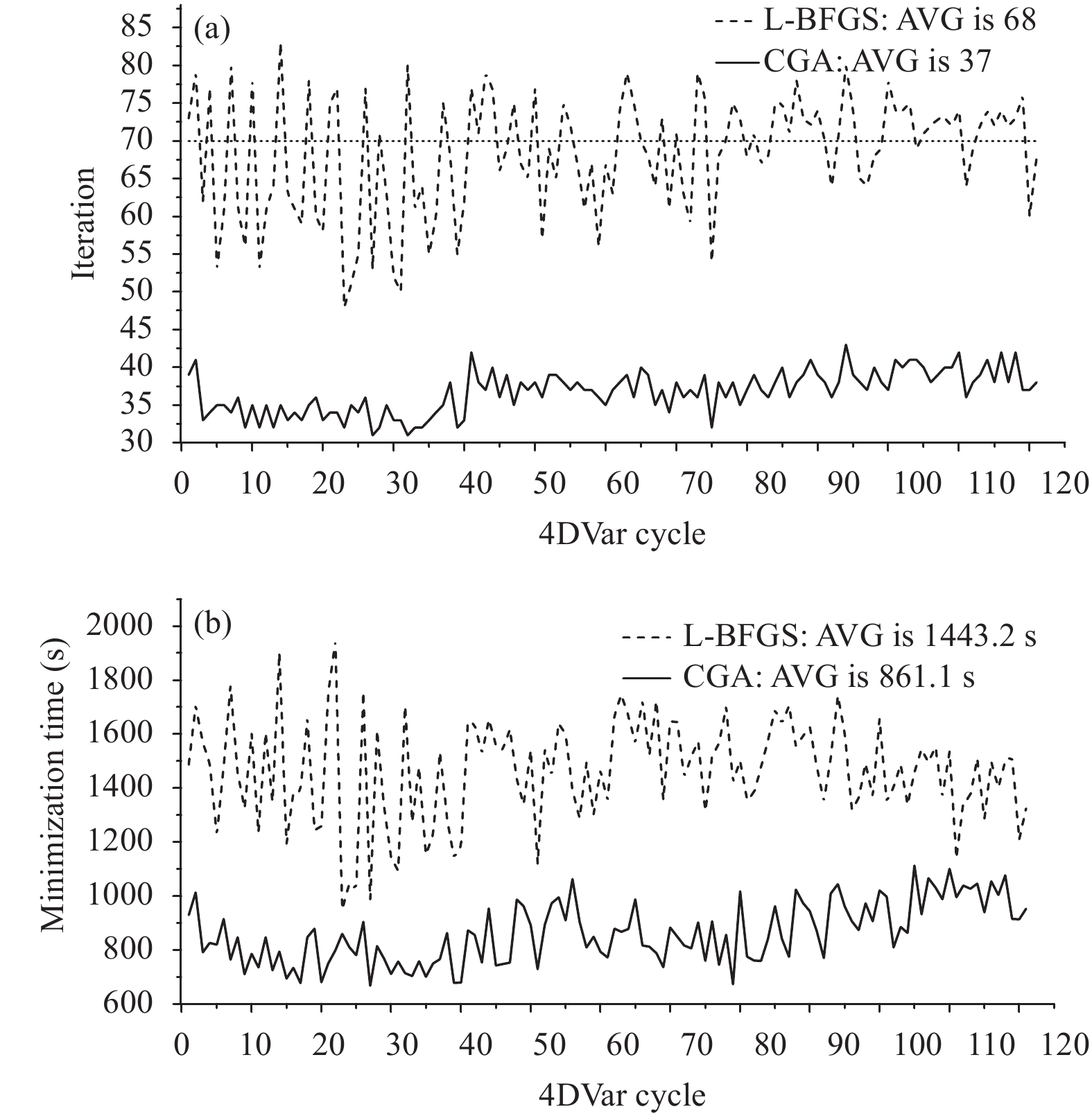Figure 6 (a) Number and (b) time of iterations in the 4DVar minimization in the 121 cyclical assimilation tests for the two experimental sets (solid line: CGA experiment; dashed line: L-BFGS experiment; AVG: the average number or time of iterations over one month)
5.3 The assimilation and forecasting results

To compare the assimilation results of the batch experiments more reasonably, we exploit the 21-day (from 10 to 30 June 2016) results of the cycling assimilation tests to avoid the influence of the initial field. A statistical analysis of the batch background and analysis deviations from the radiosonde temperature observations for the L-BFGS experiment (shown in black) and the CGA experiment (shown in red) is shown in Fig. 7. The two experiments show very similar standard deviations (Fig. 7a) and biases (Fig. 7b) of the background fields and analysis fields at all levels. In addition, the statistics of the other types of observations between the two experiments are also similar. Therefore, this shows that the estimated solutions [Eq. (1)] for these two minimization algorithms are both reasonable when they reach the same convergence rate in the 4DVar minimization (Fig. 6). This result validates the potential of using these two iterative methods in the GRAPES 4DVar minimization.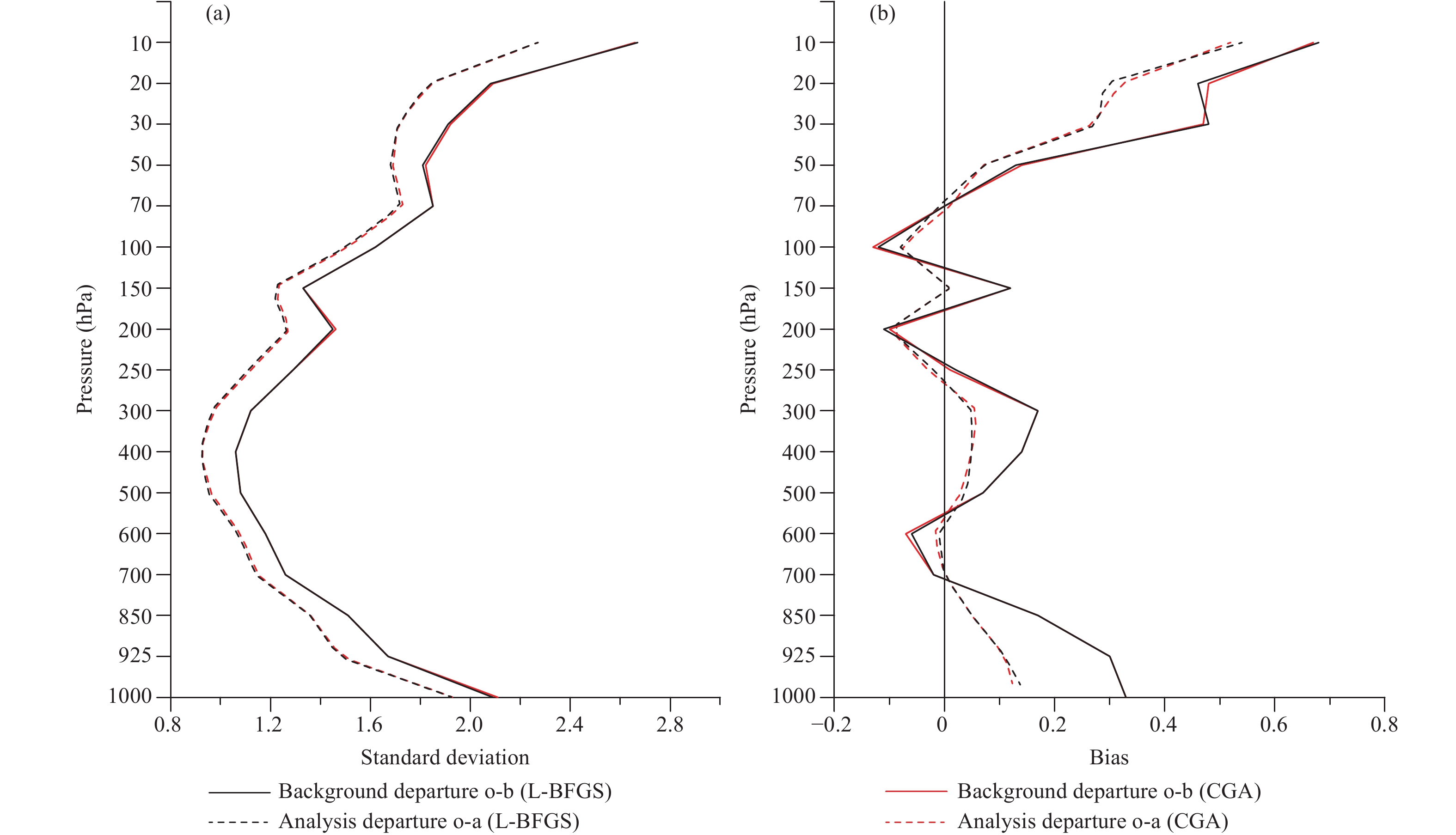Figure 7 (a) Standard deviations and (b) biases of background and analysis fields from radiosonde temperature observations for the L-BFGS experiment (black) and CGA experiment (red) (solid line: background departure o-b; dotted line: analysis departure o-a).
6 Conclusions and discussion

A CGA based on the Lanczos iteration is investigated in this paper that considers latitudinal and longitudinal grid characteristics under a non-hydrostatic framework in the GRAPES tangent linear and adjoint models. This approach solves the convergence problem through orthogonalization in the Lanczos iteration. The CGA produces equivalent analysis results with far fewer iterations and a higher computational efficiency than the L-BFGS in the batch experiments on the 4DVar system. This conclusion for the GRAPES 4DVar system is consistent with that for the ECMWF 4DVar system. However, the denser grid distribution of GRAPES adds a gradient sensitivity computed by the adjoint model, leading to an increase in the condition number of the Hessian matrix, thereby affecting the convergence rate. However, this issue can be addressed by orthogonalization. Thus, the CGA is more suitable for the operational development of the GRAPES 4DVar system, indicating that the CGA is more suitable for minimization problems such as those in the 4DVar system.

To further improve the convergence of the 4DVar minimization problem, we need to explore the preconditioned CGA based on the eigenvector of the low-resolution minimization, perform additional outer loop updates in the framework of incremental analysis, and ultimately improve the 4DVar analysis technique.

Appendix A: A description of the L-BFGS

The L-BFGS algorithm is an optimization algorithm in the family of quasi-Newton methods that employs a limited amount of computer memory. The algorithm starts with an initial estimate $\delta {{x}_0}$ , and the initial gradient of the cost function is ${{g}_0} = \nabla {J}\left({\delta {{x}_0}} \right)$ . A positive definite initial approximation of the inverse Hessian matrix is defined as E0 (which may be an identity matrix). Thus, the L-BFGS algorithm has the following basic structure for minimizing ${J}\left({\delta {x}} \right)$ (Liu and Nocedal, 1989) for ${k} = 0, 1, \cdots$ :

Step 1. Compute the search direction ${{d}_{k}} = - {{{E}}_{k}}{{g}_{k}}$ , and set $\delta {{x}_{{k + 1}}} = \delta {{x}_{k}} + {\alpha _{k}}{{d}_{k}}$ , where ${\alpha _{k}}$ is the step size obtained by a safeguarded procedure, ${{{E}}_{k}}$ is the approximation of the inverse Hessian matrix, and ${{g}_{k}} = \nabla {J}\left({\delta {{x}_{k}}} \right)$ .

Step 2. Set ${{s}_{k}} = \delta {{x}_{k}} - \delta {{x}_{{k + 1}}}$ and ${{z}_{k}} = {{g}_{k}} - {{g}_{{k + 1}}}$ . To reduce the memory usage in the algorithm, ${{{E}}_{{k + 1}}}$ is generally updated by the previous m iterations ${{s}_{k}}, {{s}_{{k - 1}}}, \cdots, {{s}_{{k - m}}}$ and ${{z}_{k}}, {{z}_{{k - 1}}}, \cdots, {{z}_{{k - m}}}$ : ${{{E}}_{{k + 1}}} = \left({{\bf{I}} - {\rho _{k}}{{s}_{k}}{z}_{k}^{\rm{T}}} \right){{{E}}_{k}}\left({\bf{I}} {- {\rho _{k}}{{z}_{k}}{s}_{k}^{\rm{T}}} \right) +$ ${\rho _{k}}{{s}_{k}}{s}_{k}^{\rm{T}}$ , where ${\rho _{k}} = {\left({{z}_{k}^{\rm{T}}{{s}_{k}}} \right)^{ - 1}}$ .

Step 3. Generate a new search direction ${{d}_{{k + 1}}} =$ $- {{{E}}_{{k + 1}}}{{g}_{{k + 1}}}$ , and then go to step 1.

The L-BFGS algorithm attempts to combine modest storage and computational requirements for minimizing ${J}\left({\delta {x}} \right)$ . Therefore, with a lower number of iterations (k < m), ${{{E}}_{{k + 1}}}$ can capture only insufficient information of the Hessian matrix, and thus, the convergence efficiency of the L-BFGS algorithm is affected.

Appendix B: A description of the CGA based on Lanczos iterations

The CGA searches in the direction of conjugated base vectors over the Krylov subspace $\mathcal{K}\left({{J''}, {{r}_0}} \right)$ and derives the minimum of the target function (Fletcher and Reeves, 1964). The equation ${{r}_0} = {b} - {J''}\delta {{x}_0}$ is the initial residual. The Lanczos approach converts the large sparse symmetric matrix into a symmetric tridiagonal matrix by an orthogonal similarity transform (Paige, 1970).

The Lanczos approach is applied on the Hessian matrix to iterate and generate a tridiagonal matrix T and an orthogonal matrix Q satisfying the relation ${{T}} = {{{Q}}^{\rm{T}}}{J''}{{Q}}$ (Golub and Van Loan, 1996). After k steps of Lanczos iterations, we generate a matrix ${{{Q}}_{k}} = \left[ {{{q}_1}, \cdots, {{q}_{k}}} \right]$ with orthonormal columns and a tridiagonal matrix ${{{T}}_{k}} = \left[ {\begin{array}{*{20}{c}} {{{\alpha }_1}}&{{{\beta }_1}}&0&0 \\ {{{\beta }_1}}&{{{\alpha }_2}}& \ddots &0 \\ 0& \ddots & \ddots &{{{\beta }_{{k} - 1}}} \\ 0&0&{{{\beta }_{{k} - 1}}}&{{{\alpha }_{k}}} \end{array}} \right]$ .

Equating the columns in ${J''}{{Q}} = {{QT}}$ , we conclude the following

 ${J''}{{q}_{k}} = {{\beta }_{{k} - 1}}{{q}_{{k - }{1}}} + {{\alpha }_{k}}{{q}_{k}} + {{\beta }_{k}}{{q}_{{k + }{1}}}.$ (B1)

The CGA based on Lanczos iterations starts with an initial estimate $\delta {{x}_0}$ ; the initial gradient of the cost function is ${{g}_0} = \nabla {J}\left({\delta {{x}_0}} \right)$ , and the initial descent direction is ${{d}_0} = - {{g}_0}$ , while the initial Lanczos vector is ${{q}_1} = {{{{{d}_0}}/{\left\| {{{d}_0}} \right\|}}_2},$ ${{\beta }_0} = 0, {{q}_0} = 0$ . We have the following basic structure for minimizing ${J}\left({\delta {x}} \right)$ (Goluband and Van Loan, 1996) for ${k} = 0, 1, \cdots$ :

Step 1. Calculate the multiplication of the matrix and vector at the kth step:

 ${{g}_{k}} = {J''}{{q}_{k}},$ (B2)

where the largest calculation lies over the whole iterative algorithm because the Hessian matrix is computed using the tangent linear model L and the adjoint model LT, the calculations of which are very large and time consuming.

Step 2. Estimate the kth diagonal element of the matrix T:

 ${{\alpha }_{k}} = \left\langle {{{g}_{k}}, {{q}_{k}}} \right\rangle .$ (B3)

Here, the notation $\left\langle { \cdots, \cdots } \right\rangle$ stands for the inner product.

Step 3. Calculate the residual vector:

 ${{r}_{k}} = {{g}_{k}} - {{\alpha }_{k}}{{q}_{k}} - {{\beta }_{{k} - 1}}{{q}_{{k - }{1}}}.$ (B4)

Step 4. Calculate the (k+1)th secondary diagonal element of the matrix T:

 ${{\beta }_{k}} = {\left\| {{{r}_{k}}} \right\|_2},$ (B5)

Step 5. Determine the Lanczos vector ${{q}_{{k + }{1}}}$ for the next iteration:

 ${{q}_{{k + }{1}}} = {{{{r}_{k}}}/{{{\beta }_{k}}}},$ (B6)

which is equivalent to the normalization of the residual vector ${{r}_{k}}$ .

Equation (B1) may be written in matrix form as follows:

 ${J''}{{{Q}}_{k}} = {{{Q}}_{k}}{{{T}}_{k}} + {{r}_{k}}{\left({{\mu _k}} \right)^{\rm{T}}},$ (B7)

where ${\left({{\mu _k}} \right)^{\rm{T}}} = \left({0, \cdots, 0, 1} \right)$ . Then, in terms of the quadratic linear equation ${{{T}}_{k}}\delta {{y}_{k}} = {{{Q}}_{k}}^{\rm{T}}{b}$ , which consists of the Lanczos matrix ${{{T}}_{k}}$ , the solution $\delta {{y}_{k}}$ of the kth step is calculated. Further, the kth approximate solution of the minimization [Eq. (1)] is estimated and associated with the Lanczos vectors $\left({{{q}_1}, \cdots, {{q}_{k}}} \right)$ .

 $\delta {{x}_{k}} = \delta {{x}_0} + \sum\nolimits_{{i} = 1}^{k} {\left\langle {{{q}_{i}}, \delta {{y}_{k}}} \right\rangle }.$ (B8)

In addition, the eigenvalues and eigenvectors of the Lanczos matrix ${{{T}}_{k}}$ can be estimated during the iteration of the Lanczos approach. The eigenvectors of ${{{T}}_{k}}$ , when pre-multiplied by ${{{Q}}_{k}}$ , approximate the eigenvectors of the Hessian matrix ${J''}$ . We can use the eigenvectors of the Hessian matrix to estimate the covariance matrix of the analysis errors because the error matrix is equal to the inversion of the Hessian matrix in the variational assimilation (Fisher, 1998). This relation can be used to precondition the CGA and improve the convergence rate.

Acknowledgments. The authors thank the editor and two anonymous reviewers for their valuable comments and suggestions in improving this manuscript.

References